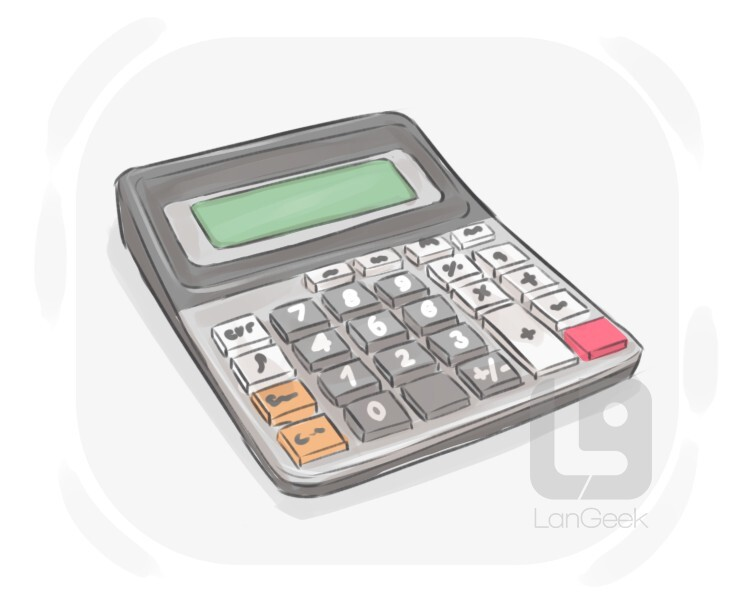# Calculator/kˈælkjʊlˌe‍ɪtɐ//ˈkæɫkjəˌɫeɪtɝ/
Calculator
[NOUN]
1

a small electronic device or piece of software used to do mathematical operations2

an expert at calculation (or at operating calculating machines)Examples
1I always keep my calculator close at hand.
2The calculator has a memory function for storing results.
3The calculator helped me quickly add up the numbers.
4They use a calculator to help with complex math calculations.
more